The MCMC Procedure
 Multivariate Density Functions

The DATA step has functions that compute the logarithm of the density of some multivariate distributions. You can use them in PROC MCMC. For a complete listing of multivariate functions, see SAS Language Reference: Dictionary.

Some commonly used multivariate functions in Bayesian analysis are as follows:

• LOGMPDFNORMAL, the logarithm of the multivariate normal

• LOGMPDFWISHART, the logarithm of the Wishart

• LOGMPDFIWISHART, the logarithm of the inverted-Wishart

• LOGMPDFDIR1, the logarithm of the Dirichlet distribution of Type I

• LOGMPDFDIR2, the logarithm of the Dirichlet distribution of Type II

• LOGMPDFMULTINOM, the logarithm of the multinomial

Other multivariate density functions include: LOGMPDFT (t-distribution), LOGMPDFGAMMA (gamma distribution), LOGMPDFBETA1 (beta of type I), and LOGMPDFBETA2 (beta of type II).

### Density Function Definition

#### LOGMPDFNORMAL

Letbe an-dimensional random vector with mean vectorand covariance matrix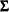. The density is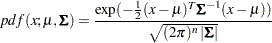where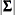is the determinant of the covariance matrix.

The function has syntax: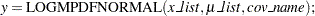Warning:you must set up thecovariance matrix before using the LOGMPDFNORMAL function and free the memory after PROC MCMC exits. See the section Set Up the Covariance Matrices and Free Memory.

#### LOGMPDFWISHART and LOGMPDFIWISHART

The density function from the Wishart distribution is: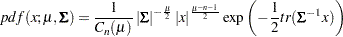with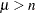, and the trace of a square matrix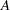is given by: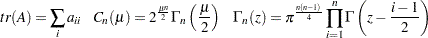The density function from the inverse-Wishart distribution is: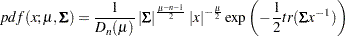for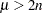, and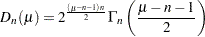If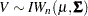then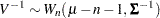The functions have syntax: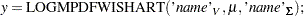and for the inverted Wishart: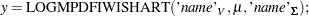The three arguments are the multivariate matrix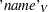, the degrees of freedom, and the covariance matrix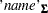k

Warning:you must set up thecovariance matrix before using these functions and free the memory after PROC MCMC exits. See the section Set Up the Covariance Matrices and Free Memory.

#### LOGMPDFDIR1 and LOGMPDFDIR2

The random variables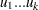, with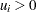and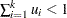, are said to have a Dirichlet Type I distribution with parameters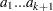if their joint pdf is given by: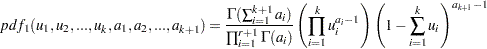The variables are said to have a Dirichlet type II distribution with parametersif their joint pdf is given by the following: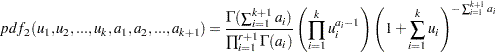The functions have syntax: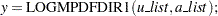and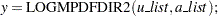#### LOGMPDFMULTINOM

Let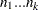be random variables that denote the number of occurring of the events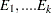respectively occurring with probabilities
. Let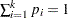and let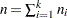. Then the joint distribution of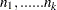is the following: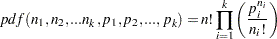The function has syntax: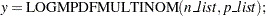### Set Up the Covariance Matrices and Free Memory

For distributions that require symmetric positive definite matrices, such as the LOGMPDFNORMAL, LOGMPDFWISHART and LOGMPDFIWISHART functions, you need to set up these matrices by using the following functions:

• Use LOGMPDFSETSQ to set up a symmetric positive definite matrix from all its elements: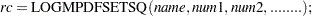is set towhen the numeric arguments describe a symmetric positive definite matrix, otherwise it is set to a nonzero value.

• Use LOGMPDFSET to set up a symmetric positive definite matrix from its lower triangular elements: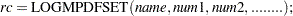When the numeric arguments describe a symmetric positive definite matrix, the returned valueis set to. Otherwise, a nonzero value foris returned.

• Use LOGMPFFREE to free the workspace previously allocated with either LOGMPDFSET or LOGMPDFSETSQ: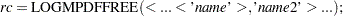When called without arguments, the LOGMPDFFREE frees all the symbols previously allocated by LOGMPDFSETSQ or LOGMPDFSET. Each freed symbol is reported back in the SAS log.

The parameters used in these functions are defined as follows:

• is a string containing the name of the work space that stores the matrix by the numeric parameters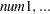.

• are numeric arguments that represent the elements of a symmetric positive definite matrix.

You would set up this matrix under the DATA step by using the following syntax: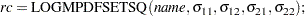or the syntax: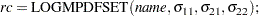If the matrix is positive definite, the returned valueis zero.Previous Page | Next Page | Top of Page